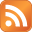# From strings to arrays to hashes and how to view the values in Perl

Published on June 4th 2019 - Listed in Perl

So I have several key/value pairs stored in a string:

my \$list = 'Reallocated_Sector_Ct=17,Uncorrectable_Error_Cnt=34000';

## From string to array

Of course I want to make an array out of these pair. The comma is used as the separator between the pairs:

my @list = split /,/, \$list;

Now the array called @list contains two values. They can be shown with a foreach loop:

foreach my \$element (@list) {
print "Aaa: \$element\n";
}

Which gives the following output:

# cat bla.pl
my \$list = 'Reallocated_Sector_Ct=17,Uncorrectable_Error_Cnt=34000';
my @list = split /,/, \$list;
foreach my \$element (@list) {
print "Aaa: \$element\n";
}

# perl bla.pl
Aaa: Reallocated_Sector_Ct=17
Aaa: Uncorrectable_Error_Cnt=34000

Another possibility is to use the data dumper module:

# cat bla.pl
use Data::Dumper qw(Dumper);
my \$list = 'Reallocated_Sector_Ct=17,Uncorrectable_Error_Cnt=34000';
my @list = split /,/, \$list;
print Dumper \@list;

# perl bla.pl
\$VAR1 = [
'Reallocated_Sector_Ct=17',
'Uncorrectable_Error_Cnt=34000'
];

The data dumper should only be used for debugging purposes.

## From array to hash

As both values in this array are a key/value pair, it makes sense to put these into a hash. The easiest method to create a hash from an existing array would be:

my %list = @list;

But this works only if the array was prepared in the following way: "key1, value1, key2, value2, key3, value3, ...".
As the array currently looks like "key1=value1,key2=value2" this won't work and results in this:

# cat bla.pl
use Data::Dumper qw(Dumper);
my \$list = 'Reallocated_Sector_Ct=17,Uncorrectable_Error_Cnt=34000';
my @list = split /,/, \$list;
my %list = @list;
print Dumper \%list;

# perl bla.pl
\$VAR1 = {
'Reallocated_Sector_Ct=17' => 'Uncorrectable_Error_Cnt=34000'
};

Not really what I wanted.

A solution for this is to go through each key value pair (through each value in our @list array), splitting up the pair using the equal sign (=) as delimiter, creating a key and a value and push them into the hash:

# cat bla.pl
my \$list = 'Reallocated_Sector_Ct=17,Uncorrectable_Error_Cnt=34000';
my @list = split /,/, \$list;
my %list;

foreach my \$pair (@list) {
print "Putting the pair \$pair into the hash\n";
(\$key, \$value) = split /=/, \$pair;
print "Look at that key: \$key\n";
print "Look at that value \$value\n";
\$list{ \$key } = \$value;
}

# perl bla.pl
Putting the pair Reallocated_Sector_Ct=17 into the hash
Look at that key: Reallocated_Sector_Ct
Look at that value 17
Putting the pair Uncorrectable_Error_Cnt=34000 into the hash
Look at that key: Uncorrectable_Error_Cnt
Look at that value 34000

Using the data dumper again, the hash looks good:

# cat bla.pl
use Data::Dumper qw(Dumper);
my \$list = 'Reallocated_Sector_Ct=17,Uncorrectable_Error_Cnt=34000';
my @list = split /,/, \$list;
my %list;

foreach my \$pair (@list) {
(\$key, \$value) = split /=/, \$pair;
\$list{ \$key } = \$value;
}

print Dumper \%list;

# perl bla.pl
\$VAR1 = {
'Reallocated_Sector_Ct' => '17',
'Uncorrectable_Error_Cnt' => '34000'
};

Or, using a for loop, going through the whole hash and print each key value pair:

# cat bla.pl
my \$list = 'Reallocated_Sector_Ct=17,Uncorrectable_Error_Cnt=34000';
my @list = split /,/, \$list;
my %list;

foreach my \$pair (@list) {
(\$key, \$value) = split /=/, \$pair;
\$list{ \$key } = \$value;
}

for my \$attribute ( keys %list ) {
my \$value = \$list{\$attribute};
print "\$attribute => \$value\n";
}

# perl bla.pl
Reallocated_Sector_Ct => 17
Uncorrectable_Error_Cnt => 34000

It's of course also possible to print a value of a single key instead of printing the whole hash:

\$ cat bla.pl
my \$list = 'Reallocated_Sector_Ct=17,Uncorrectable_Error_Cnt=34000';
my @list = split /,/, \$list;
my %list;

foreach my \$pair (@list) {
(\$key, \$value) = split /=/, \$pair;
\$list{ \$key } = \$value;
}

print "Value of Uncorrectable_Error_Cnt is: \$list{'Uncorrectable_Error_Cnt'}\n";

\$ perl bla.pl
Value of Uncorrectable_Error_Cnt is: 34000